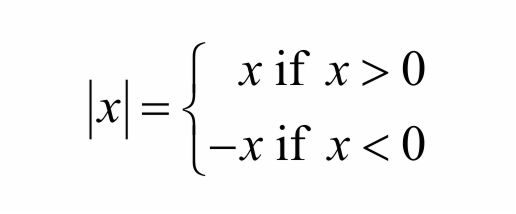# $\displaystyle \left| x \right|$, Hoplessly Positive.

Algebra Level 2If $\displaystyle { \left| x \right| }^{ 2 }+\left| x \right| -6=0$,

then which of the fowling option is true?

• (A) Product of roots is $\displaystyle -6$

• (B) Sum of the roots is $\displaystyle +1$ or $\displaystyle -1$

• (C) Both (A) and (B)

• (D) None of these.

###### AYWC?
×

Problem Loading...

Note Loading...

Set Loading...